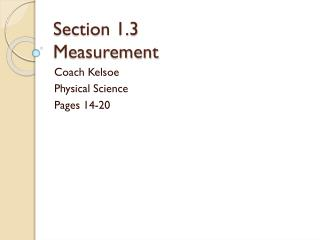DownloadDownload PresentationSection 1.3 Measurement

# Section 1.3 Measurement

Télécharger la présentation## Section 1.3 Measurement

- - - - - - - - - - - - - - - - - - - - - - - - - - - E N D - - - - - - - - - - - - - - - - - - - - - - - - - - -
##### Presentation Transcript

1. Section 1.3Measurement Coach Kelsoe Physical Science Pages 14-20

2. Objectives • Perform calculations involving scientific notation and conversion factors. • Identify the metric and SI units used in science and convert between common metric prefixes. • Compare and contrast accuracy and precision. • Relate the Celsius, Kelvin, and Fahrenheit temperature scales.

3. Using Scientific Notation • When talking about astronomically large or microscopically small numbers, it can become a hassle to count all the zeros you need to complete the number. Instead, you can use a shortcut called scientific notation. • Scientific notation is a way of expressing a value as the product of a number between 1 and 10 and a power of 10. • For example, a number like 300,000,000 would be expressed as 3.0 x 108. The exponent (8) tells you that the decimal point is really 8 places to the right of the 3.

4. Using Scientific Notation • For numbers less than 1, the exponent is negative. • For example, the number 0.00086 written in scientific notation is 8.6 x 10-4. The negative exponent tells you how many decimal places there are to the left of the 8.6. • Scientific notation makes very large or very small numbers easier to work with.

5. Using Scientific Notation • When multiplying numbers written in scientific notation, you multiply the numbers that appear before the multiplication signs and add the exponents. • For example, when multiplying 1.2 x 105 m/s by 7.2 x 103 s, the answer you would get would be 8.64 x 108 s. • When dividing numbers written in scientific notation, you divide the numbers before the multiplication sign and subtract the exponents. • For example, when dividing 8.9 x 1011 m by 4.1 x 106 m/s, the answer you would get would be 2.17 x 105 s.

6. Sample Problem • A rectangular parking lot has a length of 1.1 x 103 meters and a width of 2.4 x 103 meters. What is the area of the parking lot? • Given: length (l) = 1.1 x 103 m, width (w) = 2.4 x 103 m • Unknown: Area (A) = ?A = l x w • Solve: A = (1.1 x 103 m) x (2.4 x 103 m)A = (1.1 x 2.4) (103+3 ) (m x m)A = 2.6 x 106 m2

7. SI Units of Measurement • Measurements do not make sense unless they have a number and a unit. • Think about it – if I told you I’d give you 100 in 5, what would that mean? Dollars in minutes? Sentences in days? • Scientists use a set of measuring units called SI, or the International System of Units. • Its abbreviation comes from its original French name, which was Système International d’Unites.

8. Base Units • SI is built upon seven metric units, known as base units. • In SI, the base unit for length, or the straight-line distance between two points, is the meter (m). • The base unit for mass, or the quantity of matter in an object or sample, is the kilogram (kg).

9. Derived Units • Additional SI units, called derived units, are made from combinations of base units. Two common examples are volume and density. • Volume is the amount of space taken up by an object. • Density is the ratio of an object’s mass to its volume.

10. Metric Prefixes • The metric unit for a given quantity is not always a convenient one to use, so we use prefixes to indicate how many times the number should be multiplied or divided by 10. • You can convert between units by moving the decimal place left or right according to the unit. • Here are some of the most common prefixes:

11. Conversion Factors • The easiest way to convert from one unit to another is to use conversion factors. • A conversion factor is a ratio of equivalent measures that is used to convert a quantity expressed in one unit to another unit. 8848 meters x 1 kilometer = 8.848 kilometers 1000 meters

12. Limits of Measurement • Precision is a gauge of how exact a measurement is. • For instance, using an analog clock is the least precise, a digital clock is more precise, and a stopwatch would be the most precise. • The precision of a calculated answer is limited by the last precise measurement used in the calculation. • Accuracy is the closeness of a measurement to the actual value of what is being measured.

13. Measuring Temperature • A thermometer is an instrument that measures temperature, or how hot an object is. • There are three temperature scales that are commonly used: Fahrenheit, Celsius, and Kelvin. • °C = 5/9(°F – 32.0°) • °F = 9/5(°C) + 32.0° • K = °C + 273 • Here are the freezing point and melting point of water using the different scales:

14. Vocabulary • Scientific notation • Length • Mass • Volume • Density • Conversion factor • Precision • Significant figures • Accuracy • Thermometer